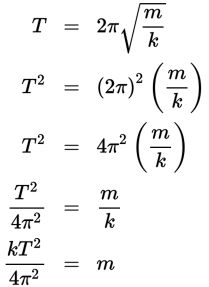# SAT Math Multiple Choice Question 440: Answer and Explanation

### Test Information

Question: 440

5.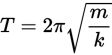When a spring is pressed tightly between two objects, it remains still. When one or both of those objects is disturbed, the spring starts to move. The equation above can be used to find the time period T in which a mass m, attached to a spring, makes a single oscillation (going all the way down and then back up). The variable k is a constant. Which of the following equations could be used to find the mass of the object?

• A.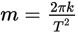• B.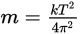• C.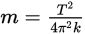• D.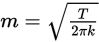Explanation:

B

Difficulty: Medium

Category: Passport to Advanced Math / Exponents

Strategic Advice: Don't spend too much time reading the scientific explanation of the equation. Focus on the question at the very end—it's just asking you to solve the equation for m.

Getting to the Answer: First, square both sides of the equation to get m out from under the radical. Then, divide both sides by 4π2. Finally, multiply both sides by k.